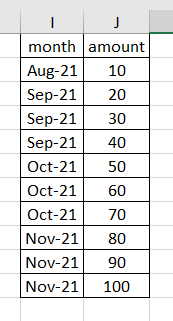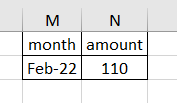# Detect year in a column

I would like to find if MMM-22 exist in the column (Don’t know how to do this without using for each. I would like to use LINQ). If no, it will sum the amount. If yes, the data table will exist as it is (I can do this).Hi @arina,

Please try the below code and let me know if it works, use an assign activity and assign the below code to a variable of type object and then you will get the output as a list so you can then check if your month exists in this list or not.

(From row in dt.AsEnumerable() Select Convert.Tostring(row(“month”)) ).ToList()

Regards.

you can see if mmm-22 exists by doing

`dt.AsEnumerable.Where(function(x) system.text.regularExpressions.regex.matches(x.Field(of string)("month"), "([A-Z][a-z][a-z]-22)").Count >= 1).Count >= 1`

@arina

Can you please share a sample input and the expected output in an excel please…that way it wpuld be easy to identify…

As of now…you can try with

Use this in if

`Dt.AsEnumerable.Where(function(x) x("month").ToString.Contains("-22")).Count>0`

Then

`Dt.AsEnumerable.Where(function(x) x("month").ToString.Contains("-22")).Sum(function(x) cdbl(x("amount")))`

On else side you have values without this as needed

Cheers

1 Like

This topic was automatically closed 3 days after the last reply. New replies are no longer allowed.